Courses

# Physics Test 12 - Unit, Dimension, Measurement, Kinematics And Laws Of Motion

## 30 Questions MCQ Test Mock Test Series for JEE Main & Advanced 2022 | Physics Test 12 - Unit, Dimension, Measurement, Kinematics And Laws Of Motion

Description
This mock test of Physics Test 12 - Unit, Dimension, Measurement, Kinematics And Laws Of Motion for JEE helps you for every JEE entrance exam. This contains 30 Multiple Choice Questions for JEE Physics Test 12 - Unit, Dimension, Measurement, Kinematics And Laws Of Motion (mcq) to study with solutions a complete question bank. The solved questions answers in this Physics Test 12 - Unit, Dimension, Measurement, Kinematics And Laws Of Motion quiz give you a good mix of easy questions and tough questions. JEE students definitely take this Physics Test 12 - Unit, Dimension, Measurement, Kinematics And Laws Of Motion exercise for a better result in the exam. You can find other Physics Test 12 - Unit, Dimension, Measurement, Kinematics And Laws Of Motion extra questions, long questions & short questions for JEE on EduRev as well by searching above.
QUESTION: 1

Solution:
QUESTION: 2

Solution:
QUESTION: 3

### The number of significant figure in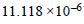V is:

Solution:
QUESTION: 4
12 coplanar forces (all of equal magnitude)maintain a body in equilibrium, then the angle between any two adjacent forces is:
Solution:
QUESTION: 5
What is the maximum number of rectangular components into which a vector can be split in space
Solution:
QUESTION: 6

Figure shows three vecotrs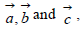where R is the midpoint of PQ. Then which of the following
relation is correct ?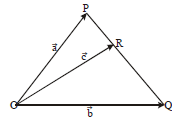Solution:
QUESTION: 7

If vectors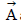and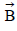are inclined to each other at angle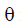then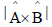is

Solution:
QUESTION: 8
If a and b are two unit vectors inclined at an angle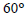to each other then
Solution:
QUESTION: 9

If a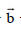c=0and |a|=1 , ||= 1 and |c|=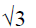then angle between a and b is

Solution:
QUESTION: 10
The angles which one the vector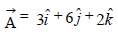makes with the coordinate axes are
Solution:
QUESTION: 11
Three vectors satisfy the relation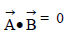and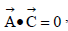then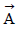is parallel to
Solution:
QUESTION: 12

a-t graph for a particle moving in a straight line is as
shown in figure. Change in velocity of the particle from t = 0 to t = 6s is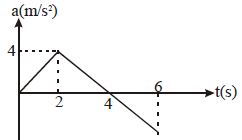Solution:
QUESTION: 13

An insect crawls a distance of 4m along north in 10seconds and then a distance of 3 m along east in 5seconds. The average velocity of the insect is

Solution:
QUESTION: 14

Let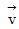and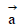denote the velocity and acceleration respectively of a particle in one dimensional motion

Solution:
QUESTION: 15
The displacement time graph of a moving particle is shown in figure. The instantaneous velocity of the particle is negative at the point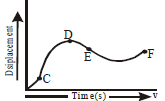Solution:
QUESTION: 16

A stone is projected with a velocity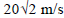at an angle of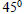to the horizontal. The average velocity of stone during its motion from starting point to its maximum height is (Take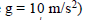Solution:
QUESTION: 17
The velocity at the maximum height of a projectile is half of its initial velocity of projection (u). Its range on horizontal plane is
Solution:
QUESTION: 18
The equation of motion of a projectile is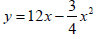Given that g =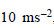what is the range of the projectile
Solution:
QUESTION: 19

Which one of the following is constant in a projectile motion ?

Solution:
QUESTION: 20

At the uppermost point of a projectile its velocity and acceleration are at an angle of

Solution:
QUESTION: 21

The maximum range of a gun on horizontal terrain is1.0 km. If g =10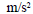what must be the muzzle velocity of the shell

Solution:
QUESTION: 22

Block B moves to the right with a constant velocity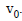The velocity of body A relative to B is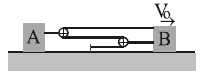Solution:
QUESTION: 23

A block of mass m is at rest on an inclined surface having a coefficient of friction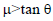as shown in the figure. The horizontal acceleration which shouldbe given to the inclined plane, that the force of friction between the block and the plane become zero, is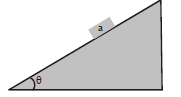Solution:
QUESTION: 24

A block of weight 5N is pushed against a vertical wall by a force 12 N. The coefficient of friction between the wall and block is 0.6. The magnitude of the force exerted by the wall on the block is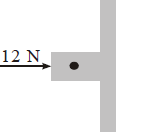Solution:

Due to normal reaction a force of 12N acts towards left.

and also as block is at rest frictional force must balance the weight. therefore frictional force is 5N in upward direction.

hence the two forces are perpendicular

so resultant = √(12*12 + 5*5 ) = 13N

QUESTION: 25

When a force F acts on a body of a mass m, the acceleration produced in the body is a. If three equal forces F1 = F2 = F3 = F act on the same body as shown in figure. The acceleration produced is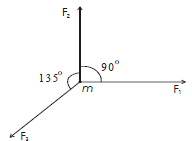Solution:
QUESTION: 26

A force vector applied on a mass is represented by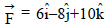and accelerates with a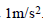What is the mass of the body

Solution:
QUESTION: 27

A monkey of mass 20 kg is holding a vertical rope.The rope will not break when a mass of 25 kg is suspended from it but will break if the mass exceeds 25 kg. What is the maximum acceleration with which the monkey can climb up along the rope ?(g=10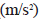Solution:
QUESTION: 28
Direction : Read the following question and choose
A. If both Assetion and Reason are true and the Reason is correct explanation of the Assertion
B. If both Assertion and Reason are true, but Reason is not correct explanation of the Assertion
C. If Assertion is true, but the Reason is false
D. If Assertion is false, but the Reason is true
Assertion : nm is not same as mN.
Reason : 1 nm =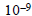m and 1m N =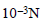Solution:
QUESTION: 29
Direction : Read the following question and choose
A. If both Assetion and Reason are true and the Reason is correct explanation of the Assertion
B. If both Assertion and Reason are true, but Reason is not correct explanation of the Assertion
C. If Assertion is true, but the Reason is false
D. If Assertion is false, but the Reason is true
Assertion : Method of dimensions cannot be used for deriving formulae containing trigonometrical ratios
Reason : This is because trigonometrical ratios have no dimensions
Solution:
QUESTION: 30
Direction : Read the following question and choose
A. If both Assetion and Reason are true and the Reason is correct explanation of the Assertion
B. If both Assertion and Reason are true, but Reason is not correct explanation of the Assertion
C. If Assertion is true, but the Reason is false
D. If Assertion is false, but the Reason is true
Assertion : The dimensional formula of surface tension is same as that for tension
Reason : Tension is force and surface tension is force per unit length
Solution: